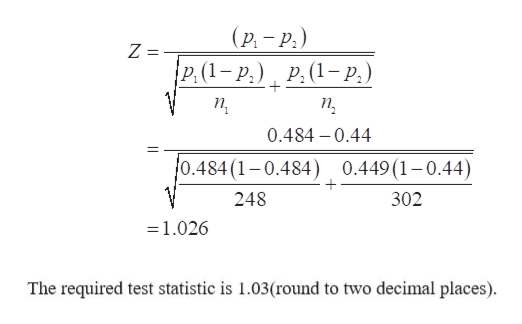# Conduct a test at thealphaαequals=0.05level of significance by determining ​(a) the null and alternative​ hypotheses, ​(b) the test​ statistic, and​ (c) the​ P-value. Assume the samples were obtained independently from a large population using simple random sampling. Test whetherp1>p2.The sample data are x1=120​,n1=248​,x2=133​,andn2=302.​(a) Choose the correct null and alternative hypotheses below.  A.Upper H 0 : p 1 equals p 2H0: p1=p2versus Upper H 1 : p 1 not equals p 2H1: p1≠p2 B.Upper H 0 : p 1 equals p 2H0: p1=p2versus Upper H 1 : p 1 less than p 2H1: p1<p2 C.Upper H 0 : p 1 equals 0H0: p1=0versus Upper H 1 : p 1 not equals 0H1: p1≠0 D.Upper H 0 : p 1 equals p 2H0: p1=p2versus Upper H 1 : p 1 greater than p 2H1: p1>p2Your answer is correct.​(b) Determine the test statistic. z0equals=nothing​(Round to two decimal places as​ needed.)

Question
20 views
Conduct a test at the
alphaαequals=0.05
level of significance by determining ​(a) the null and alternative​ hypotheses, ​(b) the test​ statistic, and​ (c) the​ P-value. Assume the samples were obtained independently from a large population using simple random sampling.

Test whether
p1>p2.
The sample data are x1=120​,
n1=248​,
x2=133​,
and
n2=302.
​(a) Choose the correct null and alternative hypotheses below.

A.
Upper H 0 : p 1 equals p 2H0: p1=p2
versus Upper H 1 : p 1 not equals p 2H1: p1≠p2

B.
Upper H 0 : p 1 equals p 2H0: p1=p2
versus Upper H 1 : p 1 less than p 2H1: p1<p2

C.
Upper H 0 : p 1 equals 0H0: p1=0
versus Upper H 1 : p 1 not equals 0H1: p1≠0

D.
Upper H 0 : p 1 equals p 2H0: p1=p2
versus Upper H 1 : p 1 greater than p 2H1: p1>p2
​(b) Determine the test statistic.

z0equals=nothing
​(Round to two decimal places as​ needed.)
check_circle

Step 1

The provided values of sample data are x1=120, n1=248 and x2=133, n2=302.

The sample proportions for both the samples can be obtained as:

Step 2

a.

The null and alternative hypothesis can be constructed as:

Null hypothesis, H0:p1= p(There is no significance difference between both the proportions).

Alternative hypothesis, H1:p1> p(The first proportion is greater than the second proportion).

Thus, option D) is correct.

Step 3

b.

The required test statistic ...help_outlineImage Transcriptionclose(р, — Р.) р.(1-р,) Р. (1-р,) п, п, 0.484 -0.44 0.484 (1-0.484) 0.449 (1-0.44) 248 302 -1.026 The required test statistic is 1.03(round to two decimal places) fullscreen

### Want to see the full answer?

See Solution

#### Want to see this answer and more?

Solutions are written by subject experts who are available 24/7. Questions are typically answered within 1 hour.*

See Solution
*Response times may vary by subject and question.
Tagged in

### Hypothesis Testing